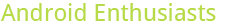4

## Please login or register to vote for this query.

(click on this box to dismiss)

"Well-Balanced" posts that exhibit both Type A and Type B behavior. These are posts that have sharp spikes of viral activity, yet are able to maintain long-term steady traffic. See comments on how to choose the "Skew Factor". For starters (SkewFactor = 4.0) is a good starting point. Anything between 1.0 and 10.0 seems to produce relevant results.Q&A for enthusiasts and power users of the Android operating system

``````-- "The Well-Balanced" - Middle-ground Type A and Type B posts from:
-- http://data.stackexchange.com/stackoverflow/query/109257/popular-posts-type-a-vs-type-b

-- Lower  "Skew Factor" favors more viral activity and less steady-state.
-- Higher "Skew Factor" favors less viral activity and more steady-state.

-- Skew Factor = 0         is the same as Type A sorting. (http://data.stackexchange.com/stackoverflow/query/109334/)
-- Skew Factor = infinity  is the same as Type B sorting. (http://data.stackexchange.com/stackoverflow/query/109312/)

declare @minDataSize numeric = 30
declare @skewFactor  numeric = ##SkewFactor?4.0##

DECLARE @endDate date
SELECT  @endDate = max(CreationDate) from Posts;

WITH rawData(type,pid,uid,age,score,vMax,vSum,vNStd)
AS(
SELECT
(CASE tmp.posttype
WHEN 1 THEN 'Q'
WHEN 2 THEN 'A'
END),
tmp.[Post Link],
tmp.[User Link],
tmp.[Age],
tmp.score,
MAX(tmp.dVotes),
SUM(tmp.dVotes),
CASE
-- If more than 30 days of votes, use standard deviation.
WHEN COUNT(tmp.dVotes) >= @minDataSize THEN STDEVP(tmp.dVotes) + 1
-- If less than 30 days of data, use some sort of zero-padded standard deviation.
ELSE
SQRT(
(
VARP(tmp.dVotes) * COUNT(tmp.dVotes)
- SQUARE(MAX(tmp.dVotes) - AVG(tmp.dVotes + 0.))
+ SQUARE(MAX(tmp.dVotes) - SUM(tmp.dVotes + 0.) / @minDataSize)
+ SQUARE(SUM(tmp.dVotes + 0.) / @minDataSize) * (@minDataSize - COUNT(tmp.dVotes))
) / @minDataSize
) + 1
END
FROM

-- Subquery forked from:
-- http://data.stackexchange.com/stackoverflow/query/108188/upvotes-per-post-and-per-day
(SELECT
p.PostTypeId AS posttype,
v.PostId AS [Post Link],
p.OwnerUserId AS [User Link],
v.CreationDate AS [Date],
DATEDIFF(DAY,p.CreationDate,@endDate) AS [Age],
Count(*) AS dVotes,
p.score AS score
FROM Votes v
LEFT JOIN Posts p ON p.Id = v.PostId
WHERE
v.VoteTypeId = 2 and p.PostTypeId is not null
GROUP BY
v.PostId,
v.CreationDate,
p.CreationDate,
p.OwnerUserId,
p.PostTypeId,
p.Score
) AS tmp

WHERE tmp.score > 0
GROUP BY
tmp.[Post Link],
tmp.posttype,
tmp.[User Link],
tmp.score,
tmp.[Age]
)

SELECT TOP 1000
type AS [ ],
pid AS [Post Link],
uid AS [User Link],
age AS [Age],
score AS [Score],
vMax AS [Vote Spike],
--    CAST(vNStd / SQRT(vSum) * 100 AS DECIMAL(20,5)) AS [Variance Index],
CAST(vNStd AS DECIMAL(20, 3)) AS [Type A],
CAST(score / vNStd AS DECIMAL(20, 3)) AS [Type B],
Cast(score /
(@skewFactor * vNStd + score / vNStd)
AS DECIMAL(20, 3)) AS [Balance]
FROM rawData
ORDER BY [Balance] DESC``````

### Enter Parameters

Options:
-Hold tight while we fetch your results
:records returned in :time ms:cached